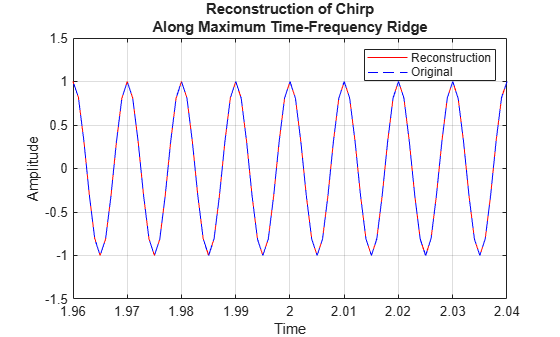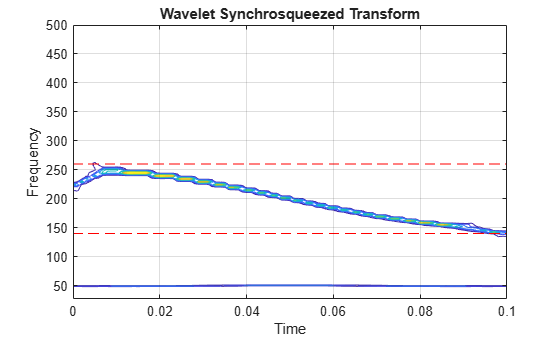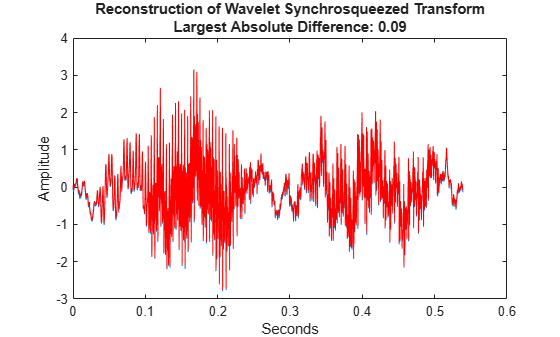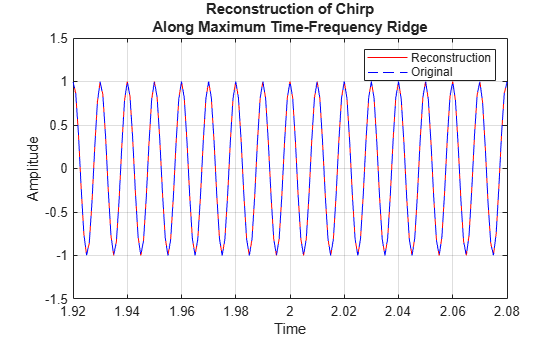Main Content

# iwsst

Inverse wavelet synchrosqueezed transform

## Syntax

``xrec = iwsst(sst)``
``xrec = iwsst(sst,f,freqrange)``
``xrec = iwsst(sst,iridge)``
``xrec = iwsst(___,wav)``
``xrec = iwsst(___,iridge,'NumFrequencyBins',numBins)``

## Description

example

````xrec = iwsst(sst)` inverts the input synchrosqueezed transform, `sst`, and returns the inverse in vector `xrec`. To obtain the `sst` input, use the `wsst` function. The `iwsst` function assumes that you obtain `sst` using the analytic Morlet wavelet. NoteThe wavelet transform does not preserve a nonzero mean. After inverting the synchrosqueezed transform, you must add back the original signal mean. ```

example

````xrec = iwsst(sst,f,freqrange)` inverts the synchrosqueezed transform for a specified range of frequencies, `freqrange`, contained in the frequency vector, `f`. The frequency vector, `f`, is the output of `wsst`.```

example

````xrec = iwsst(sst,iridge)` inverts the synchrosqueezed transform along the time-frequency ridges specified by `iridge`, the index column vector. `iridge` is the output of `wsstridge`. The `xrec` output is the same size as `iridge`.```

example

````xrec = iwsst(___,wav)` uses the analytic wavelet specified by `wav` to invert the synchrosqueezed transform. This wavelet must be the same wavelet as used in `wsst`. You can include any of the input arguments from previous syntaxes.```

example

````xrec = iwsst(___,iridge,'NumFrequencyBins',numBins)` returns the inverse synchrosqueezed transform with `numBins`-many additional frequency bins included on either side of each `iridge` index bin.```

## Examples

collapse all

Obtain the wavelet synchrosqueezed transform of a quadratic chirp using default values. Then reconstruct the signal using the inverse wavelet synchrosqueezed transform.

```load quadchirp; sst = wsst(quadchirp); xrec = iwsst(sst);```

Obtain the wavelet synchrosqueezed transform of a quadratic chirp sampled at 1000 Hz. Then reconstruct the chirp.

Load the chirp and obtain the synchrosqueezed transform.

```load quadchirp; sstchirp = wsst(quadchirp,'ExtendSignal',true);```

Extract the maximum energy time-frequency ridge and reconstruct the signal mode along the ridge.

```[~,iridge] = wsstridge(sstchirp); xrec = iwsst(sstchirp,iridge);```

Plot and zoom in on the original and reconstructed signal.

```plot(tquad,xrec,'r'); hold on; plot(tquad,quadchirp,'b--'); xlabel('Time'); ylabel('Amplitude'); set(gca,'ylim',[-1.5 1.5]); legend('Reconstruction','Original'); grid on; title('Reconstruction of Chirp Along Maximum Time-Frequency Ridge'); zoom xon zoom(50)```Obtain the inverse synchrosqueezed transform for a specified frequency range of a two-component signal. The input is a combination of an amplitude-modulated and a frequency-modulated signal.

Create the signal.

```t = 0:0.001:0.1; x1 = (2+0.5*cos(2*pi*10*t)).*cos(2*pi*200*t+10*sin(2*pi*5*t)); x2 = cos(2*pi*50*t); sig = x1+x2;```

Obtain the wavelet synchrosqueezed transform and plot the resulting two frequency components.

```[sst,f] = wsst(sig,1000,'ExtendSignal',true); contour(t,f,abs(sst)); grid on; title('Wavelet Synchrosqueezed Transform'); ylabel('Frequency'); xlabel('Time'); hold on plot(t,140*ones(size(t)),'r--'); plot(t,260*ones(size(t)),'r--');```Obtain the inverse synchrosqueezed transform for frequencies from 140 Hz to 260 Hz. Plot the result.

```xrec = iwsst(sst,f,[140,260]); subplot(2,1,1); plot(t,x1); title('Original Signal'); subplot(2,1,2); plot(t,xrec,'r'); title('Reconstructed Signal');```Obtain the wavelet synchrosqueezed transform and inverse synchrosqueezed transform of a speech sample using the bump wavelet.

Load the speech signal and obtain the synchrosqueezed transform and inverse synchrosqueezed transform.

```load mtlb dt = 1/Fs; t = 0:dt:numel(mtlb)*dt-dt; Txmtlb = wsst(mtlb,'bump'); xrec = iwsst(Txmtlb,'bump');```

Obtain the L-infinity norm of the difference between the original waveform and the reconstruction. Plot the results.

```Linf = norm(abs(mtlb-xrec),Inf); plot(t,mtlb) hold on xlabel('Seconds') ylabel('Amplitude') plot(t,xrec,'r') title({'Reconstruction of Wavelet Synchrosqueezed Transform';... ['Largest Absolute Difference: ' num2str(Linf,'%1.2f')]})```This example shows how to invert the wavelet synchrosqueezed transform using a specified number of frequency bins for a quadratic chirp. The chirp is sampled at 1000 Hz.

```load quadchirp; sstchirp = wsst(quadchirp,'ExtendSignal',true);```

Extract the maximum energy time-frequency ridge using 10 bins on each side of the iridge index and reconstruct the signal mode along the ridge.

```[~,iridge] = wsstridge(sstchirp); xrec = iwsst(sstchirp,iridge,'NumFrequencyBins',10);```

Plot the original and reconstructed signal.

```plot(tquad,xrec,'r'); hold on; plot(tquad,quadchirp,'b--'); xlabel('Time'); ylabel('Amplitude'); set(gca,'ylim',[-1.5 1.5]); legend('Reconstruction','Original'); grid on; title('Reconstruction of Chirp Along Maximum Time-Frequency Ridge');```## Input Arguments

collapse all

Synchrosqueezed transform, specified as a matrix. `sst` is the output from the `wsst` function.

Synchrosqueezed transform frequencies corresponding to the rows of the synchrosqueezed transform, specified as a vector. The number of elements in the frequency vector is equal to the number of rows in the `sst` input. If you specify `f`, you must also specify `freqrange`.

Frequency range for which to return inverse synchrosqueezed transform values, specified as a two-element vector. The values of `freqrange` must be in the range of the values of the frequencies, `f`. The first and second elements of `freqrange` define the start and end of the frequency range, where the frequency values in that range must be positive and strictly increasing. If you specify `freqrange`, you must also specify `f`.

Time-frequency ridge row indices of the synchrosqueezed transform specified as a vector or matrix. `iridge` is the output of the `wsstridge` function. If `iridge` is a matrix, `iwsst` inverts the synchrosqueezed transform along the first column of `iridge`. Then, it iteratively reconstructs along subsequent columns of `iridge`. The sizes of `iridge` and the `xrec` output are the same.

Analytic wavelet used to compute the inverse synchrosqueezed transform, specified as one of the following:

• `'amor'` — Analytic Morlet wavelet

• `'bump'` — Bump wavelet

You must use the same wavelet in the reconstruction that you used to compute the synchrosqueezed transform, `sst`.

Number of additional frequency bins to include on either side of each `iridge` index bin, specified as a positive integer. If the number of additional bins exceeds the number of frequency bins available at a particular time step, `iwsst` truncates the reconstruction at the first or last frequency bin. The default, `16`, is one half the default number of voices per octave.

To specify this argument, you also specify `iridge`, which is the output of `wsstridge`. You cannot include a frequency `f` and frequency range `freqrange`, if you include the number of frequency bins.

## Output Arguments

collapse all

Inverse synchrosqueezed transform, returned as a vector or matrix. If you do not specify an `iridge` input, `xrec` is a column vector with the same number of rows as `sst`. If you specify an `iridge` input, `xrec` is the same size as `iridge`.

 Daubechies, I., J. Lu, and H. T. Wu. "Synchrosqueezed Wavelet Transforms: an Empirical Mode Decomposition-like Tool." Applied and Computational Harmonic Analysis, Vol. 30, Number 2, 2011, pp. 243–261.

 Thakur, G., E. Brevdo, N. S. Fučkar, and H. T. Wu. "The Synchrosqueezing algorithm for time-varying spectral analysis: robustness properties and new paleoclimate applications." Signal Processing, Vol. 93, Number 5, 2013, pp. 1079–1094.

## SupportGet trial now